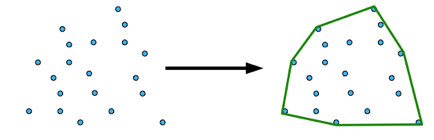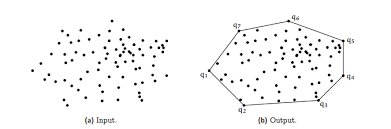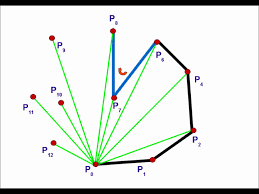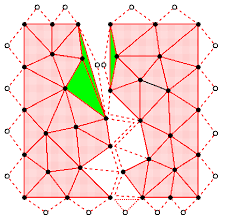convex hull :

convex hull means a hull which all given points consist in a convex polygon. All points of the hull inside the polygon and the boundary points aligned with polygon shape of geometry.In the above image some random points are given and they converted into a polygon shape and all given points is inside that geometry shape.In this image we can see some random points  inclosed in a shape and it's boundary points are q1,q2,q3,q4...,q7.

We can find convex hull using through many of Algorithms. Some Algorithms are shown below:

-> Jarvis/Wrapping Algorithm :the complexity is O(n^2) for Jarvis method. we fixed one point and compare with all points which is given in hull.

->Graham Scan Algorithm :The complexity of Graham scan algorithm is O(nlogn) times. we select a vertices point and scan all point through that select points means we apply clockwise or anticlockwise searching for next point when any value get in right side than consider otherwise accept that points. our select point change after every compare.

->Monotone chains Algorithm:

Complexity of the algorithm is O(n^3) or O(n^2.897).

->Stressen's Algorithm :

it's another name is stressen matrix chain algorithm cause Multiplications of Matrices is easily perform in that algorithm. In this algo we take two  matrix and divided into small parts than multiply them and merge it again.

->Divide and Conquer Algorithm :

It's a Greedy and Dynamics Algorithm which complexity is O(n^3).

Three steps of that algorithm:

Divide : separate all points into two part

Conquer: find two hulls through points

Merge : concatenate two parts.we will separate all given points in two part in it's lower hull and upper hull than apply brute force algorithm or grahm scan on it and after that merge it. we check it through a upper bound and a lower bound.

code in Python:

```      // define a function for hull and the value is the smallest point of vertices.
def convexhull(value):
value = sorted(set(value)) // the points which is shorted by compare in counter              clockwise order.

if len(value) <= 1:    // return positive value.         return value
// negative for clockwise turn, and zero if the points are collinear.
def cross(z, x, y):  // define a origin and coordinates point(x,y).
return (x - z) * (y - z) - (x - z) * (y - z)

// Divided the hull in two part lower and upper hull and search outer points                than merge it.
//lower hull
lower = []
for a in value:
while len(lower) >= 2 and cross(lower[-2], lower[-1], a) <= 0:
lower.pop()
lower.append(a)
//upper hull
upper = []
for a in reversed(value):
while len(upper) >= 2 and cross(upper[-2], upper[-1], a) <= 0:
upper.pop()
upper.append(a)
return lower[:-1] + upper[:-1]  // -1 means it's string in reverse .```

Application of the Algorithm :

- Video game using finger tracking.

- Gestured controller

- Finger recognition.

Resources :

- Introduction to Algorithm by Thomas H. Cormen